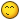# Math Question

## Recommended Posts

How does one get the cross product of two finite vectors of indeterminate dimensions.

I know how to cross 2,3,4 nth dimension vectors... but how would I write up a dynamic one for any cases?

##### Share on other sites

I'd try something like this:

``````for(var i = 0; i < numDim; i++){

r[i] = a[(i + numDim - 1) % numDim] * b[(i + numDim) % numDim];

}``````

I haven't tested this.

Edit:

``````for(var i = 0; i < numDim; i++){
r[i] = a[(i + numDim - 2) % numDim] * b[(i + numDim - 1) % numDim] - a[(i + numDim - 1) % numDim] * b[(i + numDim - 2) % numDim];
}``````

##### Share on other sites

so like:

``````Array.prototype.cross = function(a){
var x,y,z = [];
if(this.length>=a.length){x=this;y=a;}else{x=a;y=this;}
var xl = x.length;
for(var i=0; i<xl; i++){
z.push(x[(i + xl - 1) % xl] * y[(i + xl) % xl]);
}
return z;
}``````

I tried to do it with:

var arr = [0, 1, 0];
arr = arr.cross([-1,0,0]);

but that returns [0,0,0]

##### Share on other sites

In my example, a and b are the vectors that you are crossing and r is the result.

The vectors are arrays of dimensions (x is at index 0, y is at index 1, z is at index 2 and so on).  Maybe you knew this already.

##### Share on other sites

This paper http://www.unizar.es/matematicas/algebra/elduque/Talks/crossproducts.pdf  proves the following theorem

Theorem 1. Let × be a vector cross product on the vector space V . Then dimV = 1, 3 or 7.

That is vector cross products only exist in 1, 3 and 7 dimensional space.

This paper  https://arxiv.org/pdf/1310.5197.pdf generalises the result to define a cross product that works in some special vector spaces with odd dimensions.

However none of this stops you taking two n dimensional arrays and combining them in any way you wish.

##### Share on other sites

https://arxiv.org/pdf/1310.5197.pdf

Is just what I needed! thanks @JohnK.

Now to actually understand it...

##### Share on other sites

LOL thanks for the compliment, but I'm not convinced it's correct.  You might need a nested loop.  Not feeling good about the numDim -2, numDim -1 part.  Hopefully, my attempt and the paper that John supplied will be enough for you to solve the problem.

##### Share on other sites

looks like its working:
var arr = [0, 0.5, 0, 0.5]
arr = arr.cross([0, -0.8, 0, 0.2]);
returns 0,-0.2,0.8,0

and just looking that seems right.thanks
http://pryme8.github.io/plibs
I am adding a bunch of "vector" methods to the array global object as a polyfill lib.  I have not uploaded the math stuff yet and there is a ton of methods I have not put up yet, but yeah... thanks!  This one was bugging me, dot scalars, and other algebraic stuff was easy,  can I mention you provided this solution in my code?

##### Share on other sites

20 minutes ago, Pryme8 said:

can I mention you provided this solution in my code

I'd feel better about that if we could prove that it works with more dimensions.

##### Share on other sites

Check this out:

I think you are going to need another loop if you really need this to work with unlimited dimensions.

##### Share on other sites

Check this out:

I think you are going to need another loop if you really need this to work with unlimited dimensions.

That's what I thought, maybe I can test it on wolfram or something.

##### Share on other sites

``````    n = size(V,1);
k = size(V,2);
U = zeros(n,k);
U(:,1) = V(:,1)/sqrt(V(:,1)'*V(:,1));
for i = 2:k
U(:,i) = V(:,i);
for j = 1:i-1
U(:,i) = U(:,i) - ( U(:,i)'*U(:,j) )/( U(:,j)'*U(:,j) )*U(:,j);
end
U(:,i) = U(:,i)/sqrt(U(:,i)'*U(:,i));
end``````

this is a matlab solution for it.
https://en.wikipedia.org/wiki/Gram–Schmidt_process

## Join the conversation

You can post now and register later. If you have an account, sign in now to post with your account.
Note: Your post will require moderator approval before it will be visible.×   Pasted as rich text.   Paste as plain text instead

Only 75 emoji are allowed.